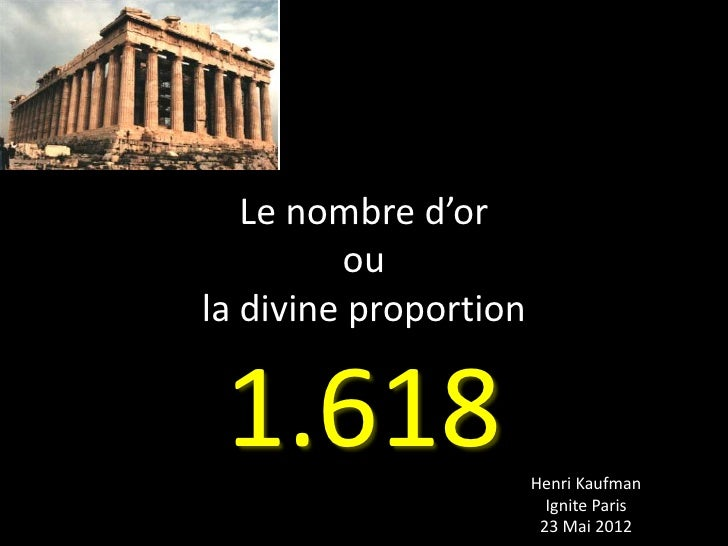Successfully reported this slideshow.Upcoming SlideShare
×

# Ignite Paris : réflexions / Nombre d&rsquo;Or

3,290 views

Published on

Le nombre d'Or (la divine proportion 1.618...) est partout dans la nature, dans le corps humain, en musique, en art... Une vidéo accompagne ces 20 slides délivrées au cours du Ignite Paris Mai 2012

Published in: Entertainment & Humor
• Full Name
Comment goes here.

Are you sure you want to Yes NoAre you sure you want to  Yes  No

Are you sure you want to  Yes  No

Are you sure you want to  Yes  No

Are you sure you want to  Yes  No

Are you sure you want to  Yes  No

### Ignite Paris : réflexions / Nombre d&rsquo;Or

1. 1. Le nombre d’or oula divine proportion 1.618 Henri Kaufman Ignite Paris 23 Mai 2012
2. 2. Le nombre d’Or1.16180339887 4989484820 …
3. 3. Définition mathématique
4. 4. Définition mathématique
5. 5. Le rectangle d’or
6. 6. De la suite à la spirale
7. 7. La suite de Fibonacci Fn = F n-1 + F n-2 1+1=2 2/1=2 1+2=3 3 / 2 = 1.5 2+3=5 5 / 3 = 1.666 5+3=8 8 / 5 = 1.6 8 + 5 = 13 13 / 8 = 1.625 13 + 8 = 21 21 / 13 = 1.615 21 + 13 = 34 34 / 21 = 1.6190 34 + 21 = 55 55 / 34 = 1.6176 55 + 34 = 89 89 + 55 = 1.61818 89 + 55 = 144 144 / 89 = 1.61789 144 + 89 = 233 233 / 144 = 1.61805 233 + 144 = 377 377 / 233 = 1.61802 377 + 233 = 610 610 / 377 = 1.61803
8. 8. Le nombre d’or et le corps humain L’homme de Vitruve Leonard de Vinci
9. 9. Le nombre d’or et le corps humain
10. 10. Nombre d’oren architecture
11. 11. Nombre d’or dans la nature
12. 12. Nombre d’or dans la nature
13. 13. Nombre d’or dans la natureLa phyllotaxie étudie la disposition des feuilles sur les tiges des plantes
14. 14. La couleur du nombre d’or
15. 15. La musique et le nombre d’or Clavier bien tempéré Jean Sébastien Bach
16. 16. Meubles aux proportions d’Or
17. 17. Sculptures aux proportions d’Or
18. 18. Sculptures aux proportions d’Or
19. 19. Le nombre d’Or en littérature Mais (0+1) Qui (1) Pourra(2) Donc faire fib (3) D’une telle contrainte (5) Sans jamais sur ses doigts compter (8) By Chrixcel
20. 20. Or’evoir !